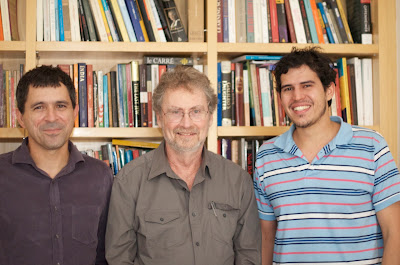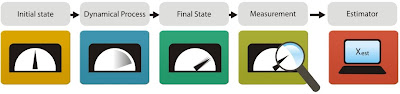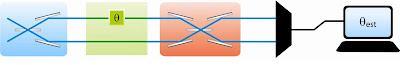# 2Physics

2Physics Quote:
"Many of the molecules found by ROSINA DFMS in the coma of comet 67P are compatible with the idea that comets delivered key molecules for prebiotic chemistry throughout the solar system and in particular to the early Earth increasing drastically the concentration of life-related chemicals by impact on a closed water body. The fact that glycine was most probably formed on dust grains in the presolar stage also makes these molecules somehow universal, which means that what happened in the solar system could probably happen elsewhere in the Universe."
-- Kathrin Altwegg and the ROSINA Team

)

## Sunday, May 29, 2011

### Quantum Metrology Meets Noise[From Left to Right] Ruynet L. de Matos Filho, Luiz Davidovich, Bruno M. Escher

Authors: Bruno M. Escher, Ruynet L. de Matos Filho, and Luiz Davidovich

Affiliation: Instituto de Física, Universidade Federal do Rio de Janeiro, Brazil

Link to the Quantum Optics and Quantum Information Group >>

The estimation of parameters is an essential part of the scientific analysis of experimental data. It may play an important role at a very fundamental level, involving the measurement of fundamental constants of Nature – as for instance the speed of light in vacuum, the Planck constant, and the gravitational constant. Furthermore, it has widespread practical implications, in the characterization of parameter-dependent physical processes – as for instance the estimation of the duration of a phenomenon, of the depth of an oil deposit through the scattering of seismic waves, of the transition frequency of an atom, or yet of the phase shift in an interferometric measurement, due to the presence of gravitational waves.

Detailed techniques for parameter estimation, dating back to the work of R. A. Fisher in 1922 [1, 2] and of H. Cramér  and C. R. Rao  a decade later, have allowed the characterization of the achievable limits in the precision of estimation. The basic steps in parameter estimation are illustrated in Figure 1: one prepares a probe in a known initial configuration, one sends it through the parameter-dependent process to be investigated, one measures then the final configuration of the probe, and from this measurement one estimates the value of the parameter.Figure 1: General protocol to estimate an unknown parameter. In the first step (first box on the left), the probe is initialized in a fiducial configuration. It is, then, sent through a parameter-dependent process, which modifies its configuration (second and third boxes). In the next step (fourth box), the probe is submitted to a measurement. Finally, an estimative of the real value of the parameter is made relying on the results of the previous measurement (last box).

Due to the fact that realistic experimental data are noisy, it is not possible to biunivocally associate an experimental result (through an estimative) with the true value of the parameter. The error in an estimative may be quantified by the statistical average of the square of the difference between the estimated and the true value of the parameter, then the so-called Cramér-Rao limit yields a lower-bound to this error, which is inversely proportional to the square root of the number N of repetitions of the measurement process. In single-parameter estimation, this bound is expressed in terms of a quantity known as Fisher Information: the larger this quantity, the more accurate can be the estimative.

Indeed, the amount of information that can be extracted from experiments about the true value of an unknown parameter is given by the Fisher Information. This quantifier of information depends on properties of the probe, the parameter-dependent process, and the measurement on the probe used to investigate the process. An important aim of metrology is to calculate the Fisher Information, to find ways to maximize it, and to find protocols that allow for better estimation.

Quantum Metrology [5, 6] takes into account the quantum character of the systems and processes involved in the estimation of parameters. In this case, the estimation error is still limited by the Cramér-Rao bound, expressed in terms of the Fisher Information, which as before quantifies the maximum amount of information that can be extracted about the parameter, but considering the constraints imposed by quantum physics; in particular, its intrinsic probabilistic nature, the dependence of the result on the measurement scheme, and the more restricted set of possible measurements.

The so-called Quantum Fisher Information  involves a maximization over all possible measurement strategies allowed by quantum mechanics. It characterizes the maximum amount of information that can be extracted from quantum experiments about an unknown parameter using the best (and ideal) measurement device. It establishes the best precision that can be attained with a given quantum probe. The ultimate precision limit in quantum parameter estimation is obtained by maximizing the Quantum Fisher Information over all initial states of the probe. In the ideal situation of systems isolated from the environment, useful analytic results allow the calculation of this ultimate bound. It can be shown then that quantum strategies, involving non-classical characteristics of the probes, like entanglement and squeezing, lead to much better bounds, as compared to standard approaches that do not profit from these properties . Thus, for a noiseless optical interferometer (Figure 2), use of entangled states of n photons leads to a precision in the measurement of phase shifts proportional to 1/n, a considerable improvement over the standard limit, which is inversely proportional to the square root of the number of photons.Figure 2: Optical interferometer. A light field, in a well-known state, is sent through the interferometer. A phase shift θ, due to a refringent material in one of the interferomter arms, can be estimated by measurements on the output field.

In practice, systems cannot be completely isolated from their environment. This leads to the phenomenon of decoherence, which mitigates quantum effects, thus limiting the usefulness of quantum strategies. In this case, it is important to establish the robustness of those strategies. However, the determination of the ultimate precision bound for systems under the influence of the environment involves usually painstaking numerical work [9, 10], since there has been up to now no general approach for this more realistic situation [11,12]. The work by Escher et al. , recently published in Nature Physics, provides a general framework for quantum metrology in the presence of noise. It can be used to circumvent this difficulty, leading to useful analytic bounds for important problems.

The main idea behind the proposed approach is to consider the probe together with an environment as a single entity, and to consider the Quantum Fisher Information corresponding to this enlarged system, which implies a maximization over all possible measurement strategies applied to the ensemble probe plus environment. This quantity is, then, an upper bound to the Quantum Fisher Information of the probe alone. It can be shown that there are several (in fact, infinite!) equivalent environments that lead to the same noisy dynamics of the probe. The work by Escher et al. shows however that it is always possible to choose an environment so that the information about the parameter, obtained from measurements on the probe plus environment, is redundant with respect to the information obtained from the probe alone. In this case, the Quantum Fisher Information of the enlarged system coincides with the corresponding quantity for the probe. This allows in principle the determination of the ultimate precision limit by using the methods previously developed for isolated systems.

Even though finding this special class of environment is in general a difficult problem, useful approximations, based on physical insights, can be found, which yield analytical bounds for the precision in noisy systems. The power of this framework was demonstrated in the paper published in Nature Physics, by applying it to lossy optical interferometry and atomic spectroscopy under dephasing, displaying in both cases how noise affects the precision in the estimation of the relevant parameter. Thus, for a noisy optical interferometer, probed by n photons, it was shown that there is a continuous transition of the precision in the estimation of phase shifts, as the number of photons increases, from a 1/n scaling, the ultimate quantum limit in the absence of noise, to the so-called standard limit, inversely proportional to the square root of the number of photons. This result shows that noise leads unavoidably to the standard limit scaling, as the number of photons reaches a critical value, which depends on the noise strength.

Due to its generality, this framework may be applied to a large variety of systems, thus offering a useful tool for the determination of the ultimate limits of precision in the estimation of parameters in realistic scenarios.

References
 R. A. Fisher, “On the mathematical foundations of theoretical statistical”, Phil. Trans. R. Soc. A, v. 222, pp. 309–368 (1922) Full text in pdf.
 R. A. Fisher, “Theory of statistical estimation”, Proc. Camb. Phil. Soc., v. 22, pp. 700–725, 1925. Abstract.
 H. Cramér, "Mathematical methods of statistics". (Princeton University Press,1946).
 C. R. Rao, "Linear statistical inference and its applications". 2nd ed. (John Wiley & Sons, 1973).
 C. Helstrom, "Quantum detection and estimation theory". (Academic, 1976).
 A. Holevo, "Probabilistic and statistical aspects of quantum theory". (North-Holland, 1982).
 S. Braunstein, C. Caves, “Statistical distance and the geometry of quantum states”, Physical Review Letters, v. 22, pp. 3439–3443 (1994). Abstract.
 V. Giovannetti, S. Lloyd, L. Maccone, “Quantum-enhanced measurements: beating
the standard quantum limit”, Science, v. 306, pp. 1330–1336 (2004). Abstract.
 S. F. Huelga, C. Macchiavello, T. Pellizzari, A. K. Ekert, M. B. Plenio, J. I. Cirac, “Improvement of frequency standards with quantum entanglement”, Physical Review Letters, v. 79, pp. 3865–3868 (1997). Abstract.
 U. Dorner, R. Demkowicz-Dobrzanski, B. J. Smith, J. S. Lundeen, W. Wasilewski, K. Banaszek, and I. A. Walmsley, “Optimal quantum phase estimation”, Physical Review Letters, v. 102, pp. 040403 (2009). Abstract.
 V. Giovannetti, S. Lloyd, L. Maccone, “Advances in quantum metrology”, Nature Photonics, v. 5, pp. 222–229 (2011). Abstract.
 L. Maccone, V. Giovannetti, “Quantum metrology: Beauty and the noisy beast”, Nature Physics, v. 7, pp. 376–377 (2011). Abstract.
 B. M. Escher, R. L. de Mattos Filho, L. Davidovich “General framework for estimating the ultimate precision limit in noisy quantum-enhanced metrology”, Nature Physics, v. 7, pp. 406–411, 2011. Abstract.